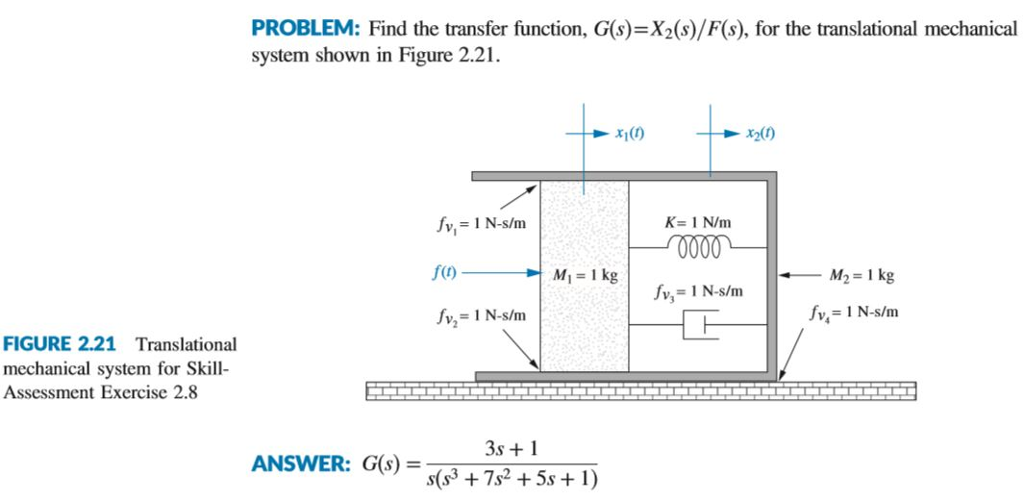### Create an Account

Home / Questions / ANSWER THE FOLLOWING QUESTIONS & DRAW THE DIAGRAM WHERE REQUIRED & WRITE EACH & EVERY...

# ANSWER THE FOLLOWING QUESTIONS & DRAW THE DIAGRAM WHERE REQUIRED & WRITE EACH & EVERY...

ANSWER THE FOLLOWING QUESTIONS & DRAW THE DIAGRAM WHERE REQUIRED & WRITE EACH & EVERY STEP AND ELABORATE EACH & EVERY STEP:-

DRAW THE FREE BODY DIAGRAM OF EACH & EVERY MASS & WRITE EACH & EVERY STEP:-PROBLEM: Find the transfer function, G(s)-X2(s)/F(s), for the translational mechanical system shown in Figure 2.2 x200 K- 1 N/m FIGURE 2.21 Translational mechanical system for Skill- Assessment Exercise 2.8 3s1 ANSWER: G(s) = s(s3 + 7s2+5s + 1)

Apr 01 2020 View more View LessSubscribe To Get Solution